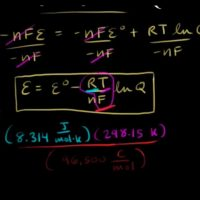# Fuel Cell Potential and Nernst equationThe Nernst equation is a depiction of the connection at other temperatures and pressures of reactants and products between the ideal standard Eo potential for the fuel cell response and the optimal E balance potential. Once the ideal potential is known under standard conditions, the ideal voltage can be determined by using these equations at other temperatures and pressures.

The Nernst equation describes how reversible voltages of electrochemical cells differ as a function of the concentration of species, gas pressure, etc. This equation is the centrepiece of thermodynamics of fuel cells.

Fuel Cell System Efficiency

A fuel cell system consists of one or more stacks of fuel cells and a number of auxiliary machinery that would also have an energy efficiency of its own. Therefore the total fuel cell system efficiency is equal to the product of the efficiency values for the fuel cell and all the auxiliary equipment.

ηs = ηFC x ηaux

In an operating fuel cell, reactant composition changes between the inlet and outlet of the fuel cell along the flow path over the electrode surface because reactant are consumed to yield current output and reaction products are formed along the way as well. The change in reactant composition results in additional loss of cell potential. This potential loss arises from the fact that the cell potential E adjusts to the lowest electrode potential given by the Nernst equation.

The Nernst equation for ideal cell potential is

E= EoRTnF ln [Reactants][Products]

Where

• R is the universal gas constant
• T is absolute temperature
• n is number of electrons involved in the chemical reaction

Steps Involved to Solve Problem using Nernst Equation

To solve problems using a Nernst equation the following steps should be taken

1. Write down the Nernst equation in symbols.
2. Determine the number of electrons released per mole of fuel oxidised (n), then determine the stoichiometric coefficients of the balanced overall cell redox reaction equation.
3. Determine the activities of all reactants or products and insert into the main equation.
4. Reduce as needed for convenience to solve.

Nernst equation has fundamental importance for analytical applications of potentiometry. The basic Nernst equation is a logarithmic relationship derived from fundamental thermodynamic equations.